# Glasser-Greene-Johnson Parameters

It is convenient to add to this appendix a generalized calculation of the magnetic curvature length,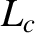, that appears in the Rutherford island width evolution equation, (12.15). Let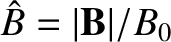,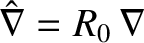, and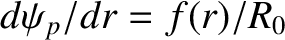. Furthermore, let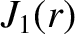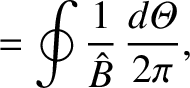(A.89)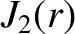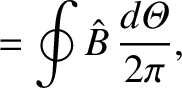(A.90)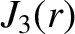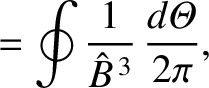(A.91)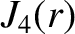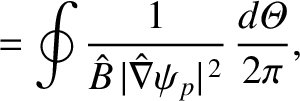(A.92)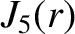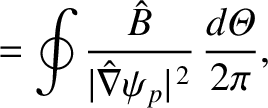(A.93)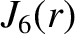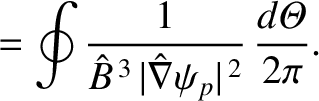(A.94)

It follows that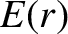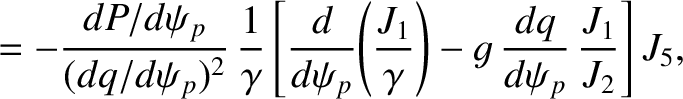(A.95)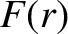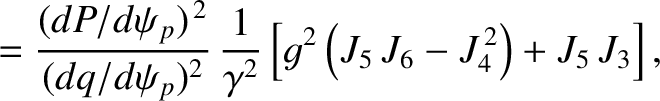(A.96)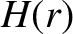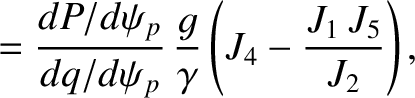(A.97)

where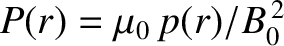, and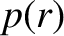is the equilibrium plasma pressure. Finally,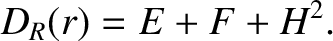(A.98)

The value of the dimensionless parameter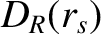at a given rational magnetic flux-surface is related to the magnetic curvature length,, introduced in Section 11.4, according to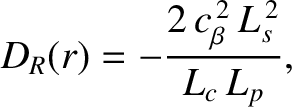(A.99)

Here,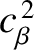is a dimensionless measure of the plasma pressure at the rational surface [see Equations (4.65) and (4.66)],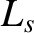the magnetic shear-length at the rational surface [see Equation (5.27)], and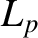the effective pressure gradient scale-length at the rational surface [see Equation (8.35)]. The previous equation is a generalization of Equation (11.57). The latter equation only holds in a large-aspect ratio, low-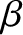, tokamak plasma with magnetic flux-surfaces of circular cross-section.